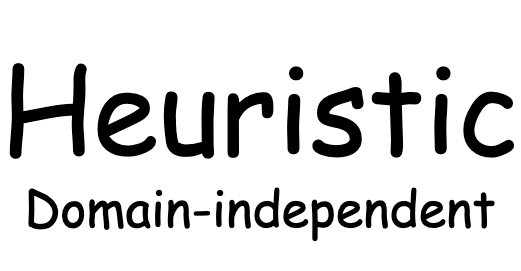# Domain-independent Heuristics

Original article was published by Debby Nirwan on Artificial Intelligence on MediumThe goal is broken down into three literals, and all of them must be True, for the Goal node to be True (Goal node is an AND node, shown by an arc connecting all its three edges).

food(a,b) = F node is an OR node, it has 3 children (let’s assume for this example), it only needs one of its children to be True. This node is an Action node.

To expand the node, we use the preconditions of the action:

• no wall in between, that is the move is possible
• Pacman is at the location

This process continues until we reach the state that we are evaluating.

The action has associated cost, after we have completed building the tree, we can calculate the cost.

Nodes in red show the state we are evaluating, the total cost of this branch is 2. Let’s assume that the next branch’s total cost is 1 and the last one is 10.

The Max-cost heuristic function returns 10 because it is the maximum cost out of three branches.

The relaxation in this approach is that it uses only the max-cost of all the literals. Planning problem is relaxed in the way that it assumes that the goal can be reached by just using one literal in the goal.

To see the difference, we use GBFS algorithm without heuristic function and with max-cost heuristic function to solve our problem.

Without heuristic, Pacman explores all possible areas as shown below.

With max-cost heuristic, Pacman is able to reduce the explored areas as shown in red dots below.

It is clear that Max-cost heuristic helps in reducing the number of nodes explored by Pacman.

In the next section we look at slightly different approach, Additive-cost Heuristic.

Additive-cost Heuristic is closely related to Max-cost heuristic. It is not admissible because the cost is often larger than the optimal cost.

Recall that 0 ≤ h(s) ≤ h*(s).

Additive-cost heuristic uses the same approach as Max-cost heuristic, but instead of choosing the largest cost among all literals, it adds all of them up. This approach is generally better in practice because it considers all literals, not only one of them.

Using And/Or Tree with cost in the picture above, the heuristic is the total of 2+1+10 = 13.

Let’s look at how it performs on our problem:

We can see that this works better than Max-cost heuristic and without heuristic and the solutions are different. See videos below to see the execution of the plans.

# Consideration

Although the number of explored nodes reduce when using these two heuristics, we need to always consider the trade-off between the computation time of heuristic function and number of reduced nodes.

A heuristic is only helpful if it reduces the total time required by search algorithm, otherwise even if it helps in reducing the number of explored nodes it is not worthwhile if the total time is more than without heuristic.

In our example, the available actions for Pacman are quite low-level in that it only moves the Pacman one step, they are not directly related to other literals in states such as foods, capsules, etc. Therefore, using these two heuristics, the total time is longer than without heuristic. Because the heuristic is essentially a backward search from goal to the state that we want to examine.

# Delete-relaxation Heuristic

In the next post we will discuss about another approach, delete-relaxation heuristic.